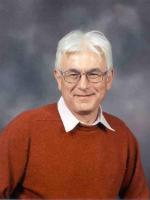# Faculty of MathematicsResearch Interests: Dynamical systems, statistical mechanics (classical and quantum), queueing networks, random processes and fields

## Publications

Large-Time Behavior of a Branching Diffusion on a Hyperbolic Space
M Kelbert, Y Suhov
– Theory of Probability and Its Applications
(2008)
52,
594
Multi-access system with many users: Stability and metastability
ND Vvedenskaya, YM Suhov
– Problems of Information Transmission
(2007)
43,
263
Loss aversion, large deviation preferences and optimal portfolio weights for some classes of return processes
K Duffy, O Lobunets, Y Suhov
– Physica A: Statistical Mechanics and its Applications
(2007)
378,
408
Anderson localisation for an interacting two-particle quantum system on ${\mathbb Z}$
V Chulaevsky, Y Suhov
On Malliavin measures, SLE and CFT
M Kontsevich, Y Suhov
Gibbs measures for SOS models on a Cayley tree
UA Rozikov, YM Suhov
– Infinite Dimensional Analysis Quantum Probability and Related Topics
(2006)
9,
471
Branching Diffusions on $H^d$ with Variable Fission: The Hausdorff Dimension of the Limiting Set
M Kelberv, YM Suhov
– Theory of Probability and Its Applications
(2007)
51,
155
A Three State Hard-Core Model on a Cayley Tree
J Martin, U Rozikov, Y Suhov
– Journal of Nonlinear Mathematical Physics
(2005)
12,
432
Malyshev's theory and JS-queues. Asymptotics of stationary probabilities
IA Kurkova, YM Suhov
– The Annals of Applied Probability
(2003)
13,
1313
The von Neumann entropy and information rate for integrable quantum Gibbs ensembles, 2
O Johnson, Y Suhov
– Quantum Computers and Computing
4,
128
• <
• 2 of 6
• >

## Research Groups

DPMMS retired
Probability
Applied Probability
Mathematical Finance and Operational Research
Statistical Laboratory

D0.24

01223 337964